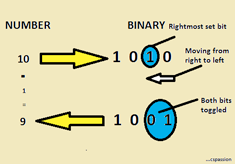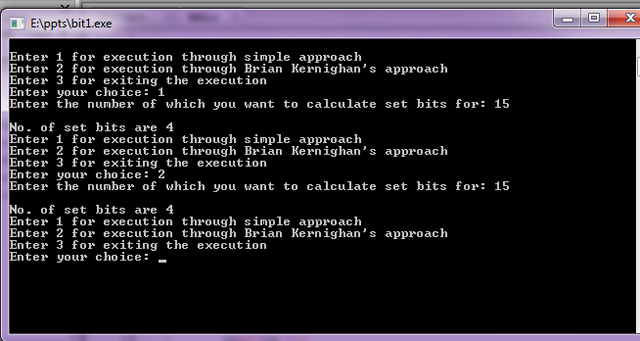## Two approaches to count the number of set bits in C

On 00:20 by Vardan Kumar in ,    No comments
Number of Set Bits

### INTRODUCTION

Set bits means the bits that are high i.e. the bits which are set to '1'.
For example:Suppose we enter '10',its binary representation is '1010',so no. of set bits in '10' are '2'.
Here we'll be discussing following two approaches -:

### 1.Simple Approach

The idea is to run the loop until the number becomes '0' after successive right shifts of the number by 1.Inside the loop we'll increment the count whenever we'll encounter 1 at LSB(Least Significant Bit). Now in order to check whether there is 1 at LSB, we'll mask it with 1(using & bit wise operation).
If applying & operation results 0 =>LSB is 0.
If applying & operation results 1 =>LSB is 1.

For example:Let us suppose we enter 10,its binary representation is 1010(Let us take 4 bits for understanding,computer usually represents a number as 32-bit binary in memory).
1.Applying '&' operation,  1 0 1 0 & 0 0 0 1=0 0 0 0 (Bit wise operation ),since we get 0=>count=0.
2.Right shift number by one,1 0 1 0 >> 1 = 0 1 0 1.
3.Applying '&' operation, 0 1 0 1 & 0 0 0 1 = 0 0 0 1(Bit wise operation),since we get 1=>count=0+1=1.
4.Right shift number by one,0 1 0 1 >> 1 = 0 0 1 0.
5.Applying '&' operation,  0 0 1 0 & 0 0 0 1 = 0 0 0 0(Bit wise operation ),since we get 0=>count=1+0=1.
6. Right shift number by one, 0 0 1 0 >> 1 = 0 0 0 1.
7.Applying '&' operation,  0 0 0 1 & 0 0 0 1 = 0 0 0 1(Bit wise operation ),since we get 1=>count=1+1=2.
8.Right shift number by one, 0 0 0 1 >> 1 = 0 0 0 0.
9.Applying '&' operation,  0 0 0 0 & 0 0 0 1 = 0 0 0 0(Bit wise operation ),since we get 0=>count=2+0=2.
10.Since number is reduced to 0,we'll exit from loop.

### 2.Brian Kernighans Approach

The idea is to subtract '1' from the number and performing bit wise '&' operation thereby.Now the question is what would subtraction get us,the answer is really simple.Observe the resulting pattern of bits and compare the same with original number.

1.Mark the first rightmost set bit.

2.Toggle each bit including the rightmost set bit while moving from right to left direction(from LSB to MSB) until you reach the marked point.

3.The resulting bits are the binary representation of  number-1.

FOR EXAMPLE....Example

We'll iterate the loop till the number becomes '0' and the number of iterations is the number of set bits.

Let us suppose we enter 15,its binary representation => 1 1 1 1
1.Now 15-1=14,its binary representation=>1 1 1 0.
2.Now 15&14 i.e. 1 1 1 1 & 1 1 1 0 = 1 1 1 0(14),count=0+1=1.
3.Now 14-1=13,its binary representation=>1 1 0 1.
4.Now 14&13 i.e. 1 1 1 0 & 1 1 0 1 = 1 1 0 0(12),count=1+1=2.
5.Now 12-1=11,its binary representation=>1011.
6.Now 12&11 i.e. 1 1 0 0 & 1 0 1 1 =1 0 0 0(8),count=2+1=3.
7.Now 8-1=7,its binary representation=>0 1 1 1.
8.Now 8&7 i.e. 1 0 0 0 & 0 1 1 1=0 0 0 0,count=3+1=4.
9.Now since the number is 0,end the iteration.
10.Return.

### SOURCE CODE

//Calculating no. of set bits of a positive number
#include<stdio.h>

unsigned int SimpleApproach(unsigned int cs)    // Complexity Theta(log(n))
{
unsigned int count=0;
while(cs)
{
count+=cs&1;
cs>>=1;
}

return count;
}
unsigned int BrianKernighans(unsigned int cs)   // Complexity O(log(n))
{
unsigned int count=0;
while(cs)
{
cs&=(cs-1);
count++;
}
return count;

}
void main()
{
unsigned int cs,passion,choice;
while(1)
{
printf("\nEnter 1 for execution through simple approach\n");
printf("Enter 2 for execution through Brian Kernighan's approach\n");
printf("Enter 3 for exiting the execution\n");
scanf("%u",&choice);
switch(choice)
{
case 1:
cs,passion=0;
printf("Enter the number of which you want to calculate set bits for: ");
scanf("%d",&cs);
passion=SimpleApproach(cs);
printf("\nNo. of set bits are %d",passion);
break;

case 2:
cs,passion=0;
printf("Enter the number of which you want to calculate set bits for: ");
scanf("%d",&cs);
passion=BrianKernighans(cs);
printf("\nNo. of set bits are %d",passion);
break;

case 3:
exit(1);

default:
printf("Wrong Choice");
}
}

}

### Output:OUTPUT
\